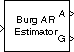# Burg AR Estimator

Compute estimate of autoregressive (AR) model parameters using Burg method

## Library

Estimation / Parametric Estimation

`dspparest3`

•## Description

The Burg AR Estimator block uses the Burg method to fit an autoregressive (AR) model to the input data by minimizing (least squares) the forward and backward prediction errors while constraining the AR parameters to satisfy the Levinson-Durbin recursion.

The input must be a column vector or an unoriented vector, which is assumed to be the output of an AR system driven by white noise. This input represents a frame of consecutive time samples from a single-channel signal. The block computes the normalized estimate of the AR system parameters, A(z), independently for each successive input frame.

`$H\left(z\right)=\frac{G}{A\left(z\right)}=\frac{G}{1+a\left(2\right){z}^{-1}+\dots +a\left(p+1\right){z}^{-p}}$`

When you select the Inherit estimation order from input dimensions parameter, the order, p, of the all-pole model is one less than the length of the input vector. Otherwise, the order is the value specified by the Estimation order parameter.

The Output(s) parameter allows you to select between two realizations of the AR process:

• `A` — The top output, A, is a column vector of length p+1 with the same frame status as the input, and contains the normalized estimate of the AR model polynomial coefficients in descending powers of z.

```[1 a(2) ... a(p+1)] ```
• `K` — The top output, K, is a column vector of length p with the same frame status as the input, and contains the reflection coefficients (which are a secondary result of the Levinson recursion).

• `A and K` — The block outputs both realizations.

The scalar gain, G, is provided at the bottom output (`G`).

The following table compares the features of the Burg AR Estimator block to the Covariance AR Estimator, Modified Covariance AR Estimator, and Yule-Walker AR Estimator blocks.

Burg AR EstimatorCovariance AR EstimatorModified Covariance AR EstimatorYule-Walker AR Estimator
`Characteristics`

Does not apply window to data

Does not apply window to data

Does not apply window to data

Applies window to data

Minimizes the forward and backward prediction errors in the least squares sense, with the AR coefficients constrained to satisfy the L-D recursion

Minimizes the forward prediction error in the least squares sense

Minimizes the forward and backward prediction errors in the least squares sense

Minimizes the forward prediction error in the least squares sense (also called “autocorrelation method”)

`Advantages`

Always produces a stable model

Always produces a stable model

`Disadvantages`

May produce unstable models

May produce unstable models

Performs relatively poorly for short data records

```Conditions for Nonsingularity```

Order must be less than or equal to half the input frame size

Order must be less than or equal to 2/3 the input frame size

Because of the biased estimate, the autocorrelation matrix is guaranteed to positive-definite, hence nonsingular

## Parameters

Output(s)

The realization to output, model coefficients, reflection coefficients, or both.

Inherit estimation order from input dimensions

When selected, sets the estimation order p to one less than the length of the input vector.

Estimation order

The order of the AR model, p. This parameter is enabled when you do not select Inherit estimation order from input dimensions.

## References

Kay, S. M. Modern Spectral Estimation: Theory and Application. Englewood Cliffs, NJ: Prentice-Hall, 1988.

Marple, S. L., Jr., Digital Spectral Analysis with Applications. Englewood Cliffs, NJ: Prentice-Hall, 1987.

## Supported Data Types

PortSupported Data Types

Input

• Double-precision floating point

• Single-precision floating point

A

• Double-precision floating point

• Single-precision floating point

G

• Double-precision floating point

• Single-precision floating point

 Burg Method DSP System Toolbox Covariance AR Estimator DSP System Toolbox Modified Covariance AR Estimator DSP System Toolbox Yule-Walker AR Estimator DSP System Toolbox `arburg` Signal Processing Toolbox

## Extended Capabilities

### C/C++ Code GenerationGenerate C and C++ code using Simulink® Coder™.

Introduced before R2006a

## Support평가판 신청